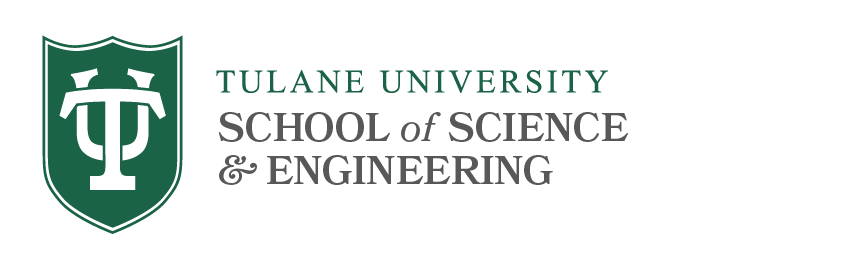# Placement Exam Syllabus

This is a 4-hour exam on topics from undergraduate courses in Linear Algebra and Advanced Calculus.

It will normally be taken at the beginning of the fall semester (the student’s first semester). If the student fails to achieve A- in the linear algebra portion, he or she will be required to enroll in Math 3090/6090. If the student fails to achieve A- in the advanced calculus portion, he or she will be required to enroll in Math 4060/6060.

## Topics Covered

### Linear Algebra

• vector spaces
• inner products
• linear transformations
• linear equations
• matrix operations
• determinants
• characteristic equation
• eigenvalues and eigenvectors
• symmetric, skew-symmetric matrices
• hermitian, skew-hermitian matrices
• Jordan canonical form
• spectral theorem

### Vector Calculus

• limits in R ⁿ
• partial derivatives
• optimization of functions in R ⁿ (with and without constraints)
• the implicit function theorem
• the inverse function theorem
• Taylor's theorem
• integration in several variables
• line integrals
• the theorems of Green, Gauss and Stokes

## Previous Exams

References you may want to study:

1. Linear Algebra, Mathematica Labs by Terry Lawson
2. Linear Algebra and Its Applications, 3rd ed., by Gilbert Strang
3. Almost any textbook with Linear Algebra in its title
4. Calculus by Boyce and DiPrima
5. Almost any textbook with Advanced Calculus in its title